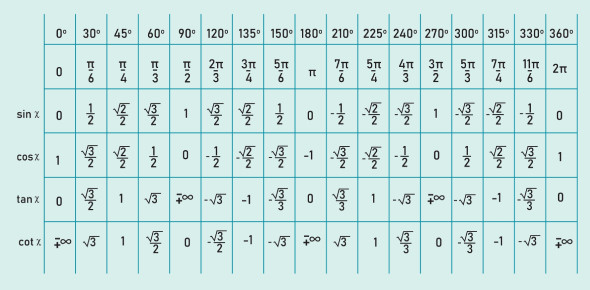# Quiz : How Much You Know About Trigonometric Domain & Range

10 Questions | Attempts: 7602
ShareSettingsHow much you know about Trigonometric Domain & Range? Try this quiz as a fun way to test your knowledge about the concepts. MCQ is a fun way to learn! Make sure to pick all the right answers to the questions given below and get a good score. We hope that the question on our quiz helps you clear your fundamental concepts and make you learn new things. Interestingly, you can attempt our quiz with your friends for excellent group preparation. Good Luck!

• 1.
The domain is the x-value in a problem.
• A.

True

• B.

False

• 2.
The range is the x-value in a problem.
• A.

True

• B.

False

• 3.
Find the domain of the point given(x, 4) when y= -8x+20.
• A.

0

• B.

2

• C.

4

• D.

6

• 4.
The ______ is the same as the cause.
• 5.
What are synonyms for the independent variable?
• A.

X, cause, output, domain

• B.

X, cause, input, domain

• C.

Y, cause, output, domain

• D.

Y, cause, input, domain

• 6.
The independent variable is the output.
• A.

True

• B.

False

• 7.
What are antonyms of an independent variable?
• A.

Output and y

• B.

Effect and range

• C.

• 8.
Find the range for the point given, (9,y), if y= -6x +63.
• A.

0

• B.

9

• C.

-9

• D.

117

• 9.
Find the domain given the range 8,4 when y= 2x -8.
• A.

2

• B.

2, 6

• C.

8

• D.

0

• 10.
The range is the independent variable.
• A.

True

• B.

False

## Related TopicsBack to top
×

Wait!
Here's an interesting quiz for you.